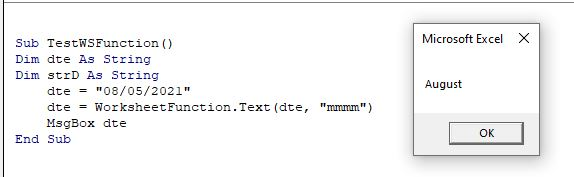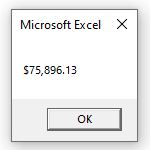# VBA Text Function

This tutorial will demonstrate how to use the Text Function in VBA.

The Text function is used in an Excel worksheet to return a portion of a string in a specified format. The Text function is not a VBA function, but can be used in VBA by referring to the Worksheet Function object.

## WorksheetFunction.Text

The syntax in VBA would therefore be as follows:

=WorksheetFunction.Text (Arg1, Arg2)

where Arg1 is the original string and Arg2 is the format of the string we want returned.

``````Sub TestWSFunction()
Dim dte As String
Dim strD As String
dte = "08/05/2021"
strD= WorksheetFunction.Text(dte, "mmmm")
MsgBox strD
End Sub``````

In the above example, the string variable dte is storing a date.  The Text function then returns the month portion of the date.## Formatting Numbers with the Text Function

We can use the text function to format numbers in our VBA code.

``````Sub FormatCurrency()
Dim strNum As String
Dim strFormat As String
strNum = "75896.125"
strFormat = WorksheetFunction.Text(strNum, "\$#,##0.00")
MsgBox strFormat
End Sub``````

The string returned in the above example would be \$75,896.13.Other examples of number formatting using the Text Function are:

``````=WorksheetFunction.Text(75896.125, "0")
this will return: "75896"

=WorksheetFunction.Text(75896.125, "0.0")
this will return: "75896.1"

=WorksheetFunction.Text(75896.125, "#,##0")
this will return: "75,896"``````

However, Excel does have a built in VBA function that we can use instead of the Text function if we wish to format dates and numbers within VBA. This is known as the Format function.

## The VBA Format Function

Taking the example above, rather than using WorksheetFunction.Text, we can just use the Format Function to achieve the same results.

``````=Format(75896.125, "0")
this will return: "75896"

=Format(75896.125, "0.0")
this will return: "75896.1"

=Format(75896.125, "#,##0")
this will return: "75,896"``````

Similarly, we  can use the Format Function to format date portions of a string.

``````Sub TestFormatFunction()
Dim dte As String
Dim strD As String
dte = "08/05/2021"
strD= Format(dte, "mmmm")
MsgBox strD
End Sub``````

Stop searching for VBA code online. Learn more about AutoMacro - A VBA Code Builder that allows beginners to code procedures from scratch with minimal coding knowledge and with many time-saving features for all users!